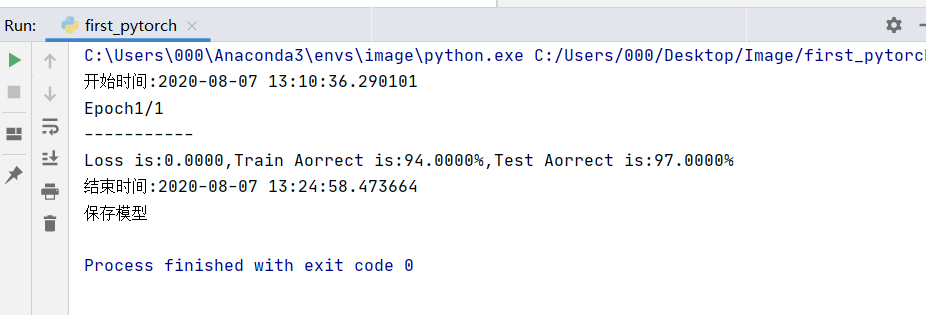• tensorflow手写数字识别python源码官网案例，带详细注释，适合刚初学tensorflow的mnist数据集训练识别， 相关链接： 手写数字识别 ----在已经训练好的数据上根据28*28的图片获取识别概率（基于Tensorflow,Python） ...
• 基于BP神经网络和sklearn的digit数据集编写的手写数字识别demo。带有GUI手写画板，同时还可以根据需要保存手写数字的数据。
• 该程序使用Python实现BP神经网络mnist手写数字识别。压缩包中给出训练好的权重以及偏移量的mat文件，在程序运行后输入mnist文件夹中图片路径的后缀就可以进行预测，如：4/mnist_test_4.png。压缩包给出训练集的mat...
• 基于knn的手写数字识别，利用python实现， 能够运行，测试集和训练集都有
• 基于卷积神经网络的手写数字识别python代码实现 卷积神经网络（Convolutional Neural Network,CNN）中，卷积层的神经元只与前一层的部分神经元节点相连，即它的神经元间的连接是非全连接的，且同一层中某些神经元...
• Neural Networks and Deep Learning的手写数字识别例程的python3版本，亲自在python shell下修改仿真后，测试无bug
• （1）认识MNIST数据集的数据格式，对...（2）利用python语言从零开始搭建多层感知机网络； （3） 通过调整参数提高多层感知机网络的准确度，并对实验结果进行评估； （4）程序的语句要求有注释，以增强程序可读性。
• 主要为大家详细介绍了python实现基于SVM手写数字识别功能，具有一定的参考价值，感兴趣的小伙伴们可以参考一下
• 使用Python基于TensorFlow 卷积神经网络设计手写数字识别算法，并编程实现GUI 界面，构建手写数字识别系统。本系统界面设计友好，功能完善。通过测试，本识别系统对于较规范的手写体数字的识别达到了很好的识别效果...
• python写的手写数字识别，建了一个CNN的神经网络
• 使用Python+TensorFlow，全连接的神经网络，基于MNIST数据集。数据集包含60000张训练图片，10000张测试图片。MNIST_model文件夹是已经训练30000次的模型，也可以自己再训练。app.py文件可以测试自己的图片。 也可以...
• 基于Matlab的手写数字识别系统，准确率还行，配有GUI界面
• 主要为大家详细介绍了机器学习python实战之手写数字识别，具有一定的参考价值，感兴趣的小伙伴们可以参考一下
• 一份基于cnn的手写数字识别的代码，供大家参考，具体内容如下 # -*- coding: utf-8 -*- import tensorflow as tf from tensorflow.examples.tutorials.mnist import input_data # 加载数据集 mnist = input_data....
• 手写数字识别,手写数字识别python,Python源码.zip
• 本文使用python基于TensorFlow设计手写数字识别算法，并编程实现GUI界面，构建手写数字识别系统。这是本人的本科毕业论文课题，当然，这个也是机器学习的基本问题。本博文不会以论文的形式展现，而是以编程实战完成...
• 在开始前已经默认你学会了，python基础 知道深度网络由那些层组成 下面的代码全部复制上可以直接运行的 如果报错，可以私聊我 训练代码 引入包 import torch from torchvision import datasets,transforms from ...
手写数字识别 python pytorch 一 之训练
下一篇：手写数字识别 python pytorch 二 之手写数字预测 https://editor.csdn.net/md/?articleId=107853422 在开始前已经默认你学会了，1 .python基础 2. 知道深度网络由那些层组成
下面的代码全部复制上可以直接运行的 如果报错，可以评论，看不懂可以评论， ** 反馈的评论修改如下： 为解决网盘老是和谐下面的链接我以把网盘资源上传到csdn上只要0积分就可以下载。系统自动给加的积分我可不是我。下面的链接可以用。 csdn下载链接点击下载 添加如下： 目录结构：慢慢人生路，相遇不易，感谢每一个点赞和评论
** 在pycharm中创建一个项目和py文件，名字随意，引入上篇文章建立的环境 上一篇：pytorch python=3.6环境安装 训练代码： 先来看怎么书写训练的代码。 引入包：
import torch
from torchvision import datasets,transforms
import numpy as np
import cv2
import datetime

没有这个包？看下面。 如果引入报错， 在该环境下base上运行（运行这个）：pip install 包名
下载数据集： 如果多次下载不成功 下载这个百度网盘链接： 里面还有本教程的全部代码在 first_pytorch文件里 有手写数字 和本教程的数据集 下载好后只要把下载的放在 和存放你所写代码 一个文件夹里就会自动调用数据集 文件名不要改！！！ 如果使用网盘下载 使用这个代码替换下面不使用网盘的代码块
transform = transforms.Compose([
transforms.ToTensor(),
transforms.Normalize((0.5,), (0.5,))
])
#数据下载
#训练数据集    是Tensor类型
data_test =  datasets.MNIST(root="./data/",transform=transform,train=False)
#测试数据集  是Tensor类型

链接：https://pan.baidu.com/s/1oihdv5ftkDfT_q7TBoQBkA 提取码：6666
transform = transforms.Compose([
transforms.ToTensor(),
transforms.Normalize((0.5,), (0.5,))
])
#数据下载
#训练数据集    是Tensor类型
data_test =  datasets.MNIST(root="./data/",transform=transform,train=False)
#测试数据集  是Tensor类型

数据装载：
#数据转载  转载包的名称  一个包中的图片数  是否打乱
data_loader_test = torch.utils.data.DataLoader(dataset = data_test , batch_size = 1, shuffle = True)

dataset参数：要装载的训练集 batch_size 参数：一匹中有多少张图片 shuffle参数：是否打乱图片顺序 搭建模型：
class Model(torch.nn.Module):#网络
def __init__(self):
super(Model,self).__init__()
self.conv1=torch.nn.Sequential(
torch.nn.ReLU(),
torch.nn.ReLU(),
torch.nn.MaxPool2d(stride=2,kernel_size=2)
)
self.dense = torch.nn.Sequential(
torch.nn.Linear(14*14*128,1024),
torch.nn.ReLU(),
torch.nn.Dropout(p=0.5),
torch.nn.Linear(1024,10)
)
def forward(self,x):
x=self.conv1(x)
x=x.view(-1,14*14*128)
x=self.dense(x)
return x

创建模型：
model = Model()
CUDA = torch.cuda.is_available()#如果你的gpu可用会自动用GPU训练
if CUDA:
model = model.cuda()#加入gpu

cost = torch.nn.CrossEntropyLoss()#损失函数

输出开始时间
start = format(datetime.datetime.now())
print("开始时间:{}".format(start))

开始训练：
epoch_n = 3#数据集训练次数 如果时间太长可以置1 我的配置显卡（gpu）2060 cpu i5-10300 运行一次时间大约十分钟
for epoch in range(epoch_n):
running_loss = 0
running_correct = 0
print("Epoch{}/{}".format(epoch+1,epoch_n))
print("-"*11)
x_train , y_train = data#标签 图片

if CUDA:
x_train = x_train.cuda()#将标签加入GPU
y_train = y_train.cuda()#将训练图片加入GPU Tensor
#-----------------------------------------------------------------------------------------------
x_train , y_train = Variable(x_train),Variable(y_train)#将Tensor 转成训练模型时 使用的数据类型

y_pred = model(x_train.data)#x_train.data 调用出图片数据                               前行传播  输出=model(模型输入)
_, pred = torch.max(y_pred, 1)#再加一层激活函数  小于一的全赋值为一
#----------------------------------------------------------------------------------------------

loss = cost(y_pred,y_train.data)#损失函数

loss.backward()#后向传播函数 通过损失函数调用
optimzer.step()#自动调优开始
#------------------------------------------------------------------------
running_loss += loss#损失值
running_correct+=torch.sum(pred == y_train.data)#成功率
testing_correct =0
x_test , y_test = data
if CUDA:
x_test = x_test.cuda()
y_test = y_test.cuda()
x_test, y_test = Variable(x_test),Variable(y_test)
y_pred = model(x_test.data)
_, pred = torch.max(y_pred,1)
testing_correct +=torch.sum(pred == y_test.data)
print("Loss is:{:.4f},Train Aorrect is:{:.4f}%,Test Aorrect is:{:.4f}%".format(running_loss//len(data_train),
100*running_correct//len(data_train),100*testing_correct//len(data_test)))
if CUDA:
torch.cuda.synchronize()#cpu 和GPU 同步 防止异步出现错误

保存模型
print("保存模型")#保存训练好的参数 下次使用直接调用不用训练
state = {'conv1':model.conv1.state_dict(),'dense':model.dense.state_dict(),}
torch.save(state,'models.pkl')#把参数写入model.pal文件

‘conv1’:神经网络训练参数，下次直接加载不用训练了 ‘dense’：神经网络结构。 结束时间
end = format(datetime.datetime.now())
print("结束时间:{}".format(end))

输出结果 只要保存好模型这个代码就测试成功，输出只不过是为了看一下损失值。这个数据集已经被预处理过我们不需要预处理。 调用GPU是为了加快训练速度，没有GPU也可以训练，速度会非常慢 我的GPU是2060 的训练一轮需要20分左右 loss is ： 损失值就是预测结果与实际值的差值 Train Aorrect is :训练成功率100张图片有50张训练时预测成功就是50% Text Aorrect is :测试成功率100张图片有50张预测成功就是50%
输出结果图片： 由于我是演示所以只训练一轮下一篇：手写数字识别 python pytorch 二 之手写数字预测https://editor.csdn.net/md/?articleId=107853422
展开全文深度学习
• 手写数字识别,手写数字识别python,Python源码.rar.zip
• python实实现现基基于于SVM手手写写数数字字识识别别功功能能 这篇文章主要为大家详细介绍了python实现基于SVM手写数字识别功能文中示例代码介绍的非常详细具有一 定的参考 值感兴趣的小伙伴们可以参考一下 本文实例...
• python语言编写，利用TensorFlow建立两层卷积神经网络，数据集为手写识别数据集MNIST，识别准确率99%
• 预处理手写数字函数： def shouxiefix(path_tu): #path_tu = 'D:\\002.png' img = cv2.imread(path_tu,0)# 蓝95 绿200 红147通道数(-1) 数组 高 宽 通道459 362 3 img = cv2.resize(img,(28,28)) """cv2.imshow...
手写数字识别 python pytorch 二 之手写数字预测
上一篇： 手写数字识别 python pytorch 一 之手写数字预测 直接在上一篇代码下复制或书写 本片和上一篇代码直接复制即可运行 注意 手写数字必须在图片中间，预测效果才好 预处理手写数字函数： imread（）
def shouxiefix(path_tu):
#path_tu = 'D:\\002.png'
#这个函数可以看我这篇文章的详细介绍 ref  https://blog.csdn.net/weixin_44021118/article/details/107769561)
img = cv2.imread(path_tu,0)#  蓝95 绿200 红147通道数(-1)   数组    高 宽 通道459 362 3

img = cv2.resize(img,(28,28))
"""cv2.imshow('img',img)#查看读入图片

cv2.waitKey(0)#按任意键继续
cv2.destroyAllWindows()#关闭图片窗口"""
#gao=img.shape
#kuan=img.shape
gao=28#指定
kuan=28#
img=cv2.resize(img,(kuan,gao))#看图片上的 kuan gao
#tongdao=img.shape
img = img.astype(np.float32)
#进行归一化
if img>100:#如果是白底黑字 转成黑底白字 并进行归一化
for i in range(gao):#tensor 蓝95 绿200  红  147 ->红 绿 蓝
for j in range(kuan):
if img[i][j]<100:
img[i][j] = 0.95
else:
img[i][j] = -1
else:#否者 进行归一化
for i in range(gao):#tensor 蓝95 绿200  红  147 ->红 绿 蓝
for j in range(kuan):
if img[i][j]>100:
img[i][j]=0.95
else:
img[i][j]=-1
"""cv2.imshow('img2',img)#查看归一化后的图片
cv2.waitKey(0)#按任意键继续
cv2.destroyAllWindows()"""

torch_img = torch.from_numpy(img)#将ndarray 数组转成Tensor
torch_img = torch .squeeze(torch_img)#函数可以删除数组形状中的单维度条目
torch_img = torch_img.unsqueeze(0)#给第0维添加一个维度变为三维
torch_img = torch_img.unsqueeze(0)#给第0维添加一个维度变为四维
torch_img=torch_img.float()#将unit8类型转为float32


读取模型
model.conv1.load_state_dict(torch.load('model.pkl')['conv1'])

测试代码
#验证单个图片
#输入 使用cpu预测

#model = model.cpu()
x_test=shouxiefix(path_tu= '006.png')#图片地址'006.png' 和这个文件一个文件夹
#x_test = x_test.cpu()
x_test = Variable(x_test)#将Tensor 转为 模型输入类型
y_pred = model(x_test.data)
_, pred = torch.max(y_pred,1)
#print(y_pred)
print(int(pred))

输出预测结果 6 输入图片展开全文深度学习
• 手写数字识别,手写数字识别python,matlab源码.rar
• 手写数字识别,手写数字识别python,matlab源码.zip...

# 手写数字识别pythonpython 订阅